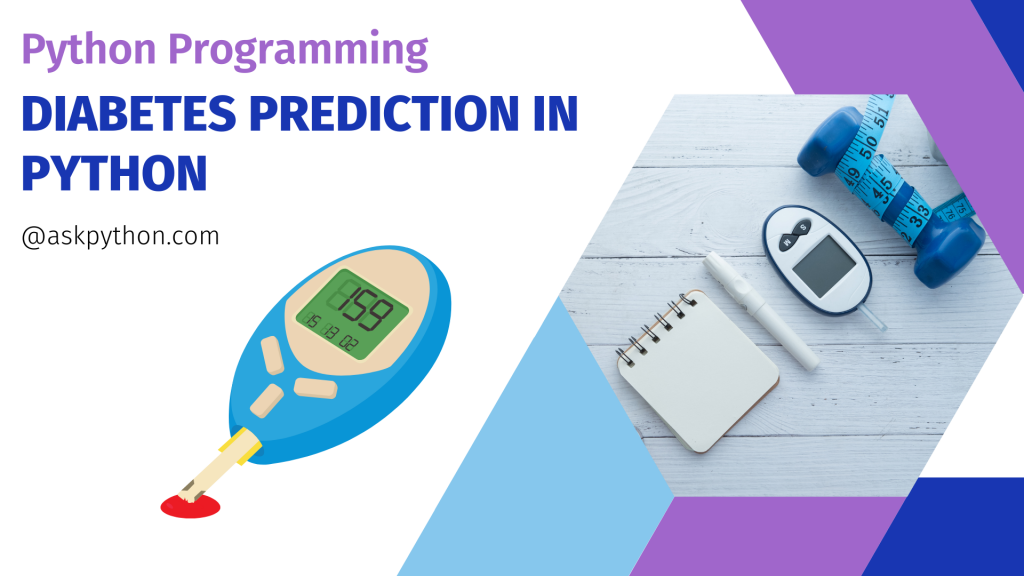# Diabetes Prediction in Python – A Simple GuideHey folks! In this tutorial, we will learn how to use Keras’s deep learning API to build diabetes prediction using deep learning techniques in Python.

## Implementing the Diabetes Prediction in Python

We will leverage an available dataset for this purpose, and we will build a deep neural network architecture. The dataset is available for download here.

You may study the dataset after downloading it, and you will notice that it is separated into 0’s and 1’s. Let’s go on to implementing our model in Python with TensorFlow and Keras.

I hope you have already installed all of the libraries on your local system. If not, no worries, you may open Google Colab and practice this lesson with me.

### Step 1 – Importing Modules

Now, let’s import the necessary Python libraries into our notebook.

Keras API already includes Python’s TensorFlow deep learning package, which is critical in the diabetes prediction challenge.

```import numpy as np
import pandas as pd
import tensorflow as tf
from keras.layers import Dense,Dropout
from sklearn.model_selection import train_test_split
import matplotlib as mlp
import matplotlib.pyplot as plt
%matplotlib inline
from sklearn.preprocessing import StandardScaler
```

We are now ready to begin importing the dataset. In the next piece of code, we import the dataset and use the head() method to get the top five data points.

```data=pd.read_csv("pima-indians-diabetes.csv")
```

### Step 3 – Renaming the Columns

You’ve probably realized that the columns are meaningless, right? Let us now rename the column names.

```data = data.rename(index=str, columns={"6":"preg"})
data = data.rename(index=str, columns={"148":"gluco"})
data = data.rename(index=str, columns={"72":"bp"})
data = data.rename(index=str, columns={"35":"stinmm"})
data = data.rename(index=str, columns={"0":"insulin"})
data = data.rename(index=str, columns={"33.6":"mass"})
data =data.rename(index=str, columns={"0.627":"dpf"})
data = data.rename(index=str, columns={"50":"age"})
data = data.rename(index=str, columns={"1":"target"})

```

### Step 4 – Separating Inputs and Outputs

```X = data.iloc[:, :-1]
Y = data.iloc[:,8]
```

The X and Y values look somewhat like this:

We separated our dataset into input and target datasets, which implies that the first eight columns will serve as input features for our model and the last column will serve as the target class.

### Step 5 – Train-Test Split of the Data

The next step involves the training and testing split into data and then standardizing the data to make computations simpler later on.

```X_train_full, X_test, y_train_full, y_test = train_test_split(X, Y, random_state=42)
X_train, X_valid, y_train, y_valid = train_test_split(X_train_full, y_train_full, random_state=42)
```
```from sklearn.preprocessing import StandardScaler
scaler = StandardScaler()
X_train = scaler.fit_transform(X_train)
X_valid = scaler.transform(X_valid)
X_test = scaler.transform(X_test)
```

### Step 6 – Building the Model

We start off by using a random seed to generate a pseudo-random number and setting it to the tf graph. Then, we will be using a sequential model, and also some dropout layers in the model to avoid overfitting of the data.

```np.random.seed(42)
tf.random.set_seed(42)

model=Sequential()
```

### Step 7 – Training and Testing of the Model

Now, let’s move forward to train our model and then fit the model on the testing dataset.

```model.compile(loss="binary_crossentropy", optimizer="SGD", metrics=['accuracy'])
model_history = model.fit(X_train, y_train, epochs=200, validation_data=(X_valid, y_valid))
```

You will realize that will train the model for 200 epochs and use binary-cross entropy loss function and SGD optimizer.

## Conclusion

Congratulations! You just learned how to build a Diabetes Predictor using the Python programming language. Hope you enjoyed it! 😇

Liked the tutorial? In any case, I would recommend you to have a look at the tutorials mentioned below:

Thank you for taking your time out! Hope you learned something new!! 😄